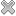# Courses / Module

Toggle Print

##STATISTICAL METHODS FOR DATA SCIENCE

Module code: ST863D
Credits: 10
Semester: 1
Department: MATHEMATICS AND STATISTICS
International:Overview

This course covers probability and statistical techniques for data analytics and data science. Topics include: Exploratory data analysis and visualisation. Probability basics, independence, Bayes theorem. Probability models for data, including Binomial, Poisson, Exponential and Normal. Parameter estimation; method of moments and maximum likelihood. Confidence intervals and hypothesis testing: one and two samples, paired samples, proportions. Simple linear and multiple regression. Analysis of Variance. Case studies in R.

Lectures and labs as for ST663.Learning OutcomesTeaching & Learning methodsAssessmentRepeat optionsPre-RequisitesTimetableV5.2.0 - Powered by MDAL Framework © 2019